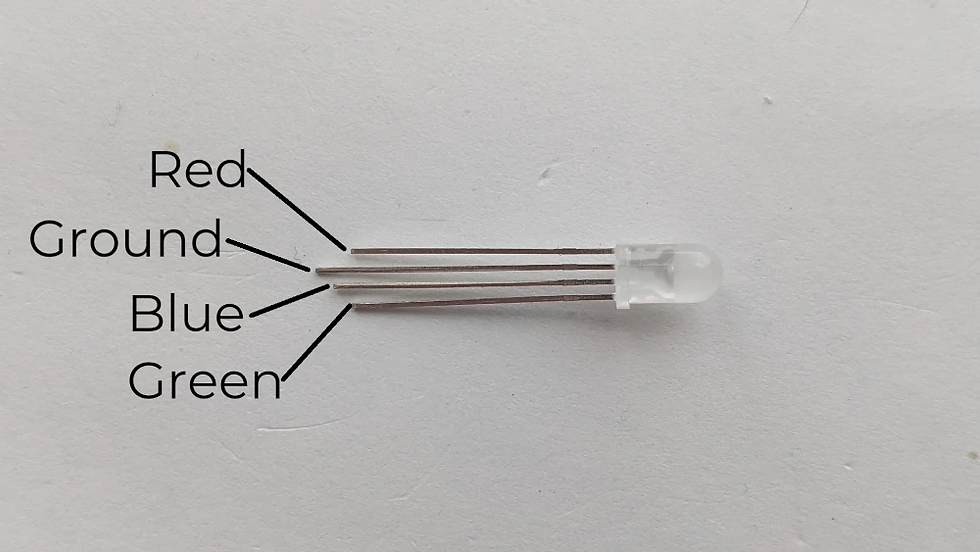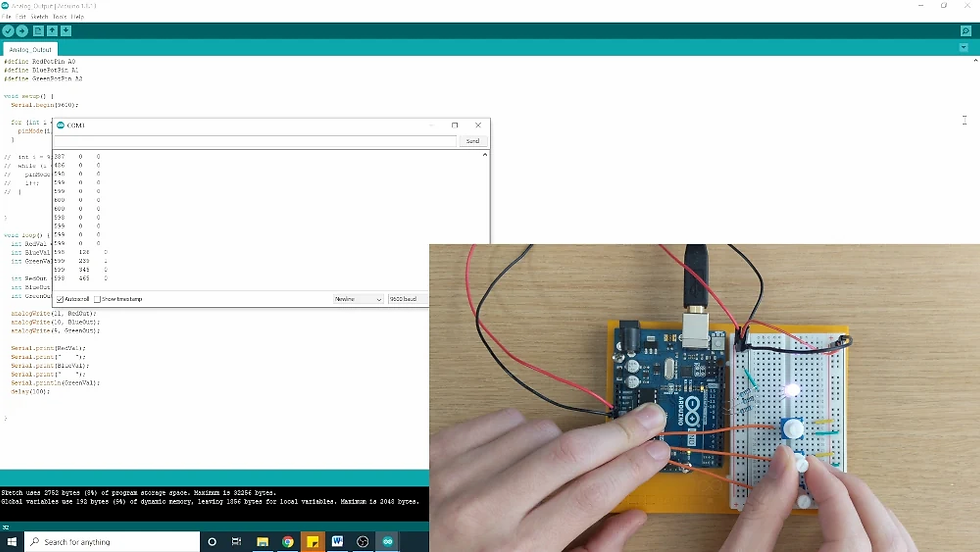top of page
Search

# How to Create an Analog Signal with Arduino

Updated: Jan 2, 2022

In this next post in the Introduction to Arduino series, I will show how to output a "fake" analog signal coming from the digital pins on the Arduino.

You can create an analog signal from the Arduino using something called pulse width modulation, or PWM. PWM is a type of digital signal that alternates from high to low at a uniform frequency. Arduino's PWM output is about 490Hz. For PWM signal, the duty cycle represents the percentage of time that the signal in the "High" state. Therefore, a 50% duty cycle from a pin that is alternating from 0V to 5V would create a signal that is perceived as 2.5V. If the duty cycle is 25%, then measured voltage would be about 1.25V.On the Arduino Uno, only the digital pins with the "~" symbol next to the number are capable of outputting a PWM signal.Parts Needed

For this example you will need:

• an Arduino Uno

• RGB LED,

• three 220Ω resistors,

• three potentiometers,

• and jumper wires.

Building the Circuit

This circuit is intended to have the Arduino read values from three different potentiometers while sending analog values to the leads of an RGB LED. These analog output values will be depend on the values being read from the potentiometers.

• Wire 5V and ground to the power bus rails on the breadboard.

• Place the RGB LED in the breadboard. The leads are close together, so you may have to bend them out.

The RGB LED has four leads. The longest lead is the cathode of the LED. The other leads direction control how much red, blue, or green are being emitted. The diagram below, describes what each of the leads does.• Connect the ground to the cathode of the LED.

• Wire the 220Ω resistors from digital pins 9, 10, and 11 to the anodes of the LED.

• Place the potentiometers on the breadboard.

• Connect the outputs of the three potentiometers to analog input pins A0, A1, and A2.

• Then connect 5V and ground to the potentiometers.Arduino Code

The goal of this sketch is to read analog values from the potentiometers, manipulate these values, and use them to output PWM signals from the digital pins. This will ultimately light up the RGB LED. The Arduino sketch can be found here.

• Start by creating three variables to assign analog pins A0-A2.

```#define RedPotPin A0
#define BluePotPin A1
#define GreenPotPin A2```
• In the setup, set the baud rate to 9600.

`Serial.begin(9600);`
• Set digital pins 9-11 using a "for loop.

• For the "for" loop, the first argument initializes a variable, the second argument is the condition that has to be met to exit the loop, and the third argument iterates the variable. Within the loop are the commands to are to be executed after each iteration.

```for (int i = 9; i <= 11; i++){
pinMode(i, OUTPUT);
}```
• A while loop can achieve the same thing. The difference is that the initialized variable will be before the while loop, the exit condition is the only argument, and the instructions and iterator are inside of the loop.

```int i = 9;
while (i <= 11){
pinMode(i, OUTPUT);
i++;
}```
• Either the "for" loop or "while" loop can be used. Using both of them in this sketch is redundant.

• In the loop, create variables that will read the analog values for the three potentiometers.

```int RedVal = analogRead(RedPotPin);
• Then use the "map" function to covert values that are within a range of 0-1024 to 0-255. The argument for the "analogWrite" commands only accepts values from 0-225.

```analogWrite(11, RedOut);
analogWrite(10, BlueOut);
analogWrite(9, GreenOut);```
• Lastly, print out any values that you want to read.

```Serial.print(RedVal);
Serial.print("    ");
Serial.print(BlueVal);
Serial.print("    ");
Serial.println(GreenVal);
delay(100);```

After the upload, you should be able to see that rotating the knob on each of the potentiometers will lower or raise the amount of red, green, and blue being emitted from the LED.Thanks for following along. If you would like to stay up to date with this Introduction to Arduino Series, please subscribe below.

The Bored Robot LLC is a participant in the Amazon Services LLC Associates Program, an affiliate advertising program designed to provide a means for sites to earn advertising fees by advertising and linking to Amazon.com.